﻿ 基于吸引子特征量的非线性判定法在大坝位移监测数据时间序列中的应用

# 基于吸引子特征量的非线性判定法在大坝位移监测数据时间序列中的应用Application of Nonlinear Decision Method Based on Attractor Characteristic Quantity in Time Series of Dam Displacement Monitoring Data

Abstract: Because of the noise of dam displacement monitoring data, a non-linear judgment method based on attractor characteristic is introduced to solve the accuracy of non-linear judgment under high noise level. In this paper, the test performance of two kinds of nonlinear chaotic time series, Lorenz equation and Henon map, is compared and analyzed when the time inversion irreversibility and attractor characteristic are used as feature statistics respectively under different noise dis-turbances. It is considered that the surrogate data method with Hurst exponent as the non-linear characteristic shows strong robustness. It is applied to the time series of displacement monitoring data of three dam observation points in practical engineering. The results show that the dis-placement time series of dam monitoring data presents the characteristics of non-linear change. This lays the foundation for analyzing the change of dam structure and grasping the operation state of the dam in time.

1. 引言

2. 吸引子特征量

1) Lyapunov指数

$\lambda =\mathrm{lim}\frac{1}{n}{\underset{i=0}{\overset{n-1}{\sum }}\mathrm{ln}|\frac{\text{d}F\left(x\right)}{\text{d}x}|}_{x={x}_{i}},\left(i=1,2,\cdots ,n\right)$ (1)

2) 分形维数

“分形”的特征就是具有自相似性，是描述混沌运动的几何语言。人们通过定义容量维，Huasdorff维、盒维数、信息维和关联维等分形维数来表征分形，本文主要计算关联维数。

$\underset{r\to 0}{\mathrm{lim}}{C}_{XX}\left(\epsilon \right)\propto {\epsilon }^{{D}_{2}}$ (2)

3) Kolmogorow熵

Kolmogorov熵描述的是混沌轨道随时间演化信息的产生率。当Kolmogorov熵大于0，且为常量时，该系统是混沌系统。采用关联积分法计算，

${K}_{2}=-\underset{\tau \to 0}{\mathrm{lim}}\underset{r\to 0}{\mathrm{lim}}\underset{m\to \infty }{\mathrm{lim}}\frac{1}{m\tau }\mathrm{ln}C\left(r,m\right)$ (3)

${K}_{2}=\frac{1}{m\tau }\mathrm{ln}\frac{{r}^{{D}_{2}}}{C\left(r,m\right)},r\to 0$ (4)

4) Hurst指数

Hurst指数是英国水利专家H. E. Hurst在1951年提出的一种判别时间序列是否对于时间有依赖的参数。任意在时间序列中取一个长度为n的时窗，求取该时窗内的数据均值 ${\stackrel{¯}{y}}_{t}$ ，计算相应的累积偏差A(t, n)。将同一个时窗n内累积偏差的最大值和最小值之差称为域，记为

$R\left(t,n\right)=\mathrm{max}A\left(t,n\right)-\mathrm{min}A\left(t,n\right)，\left({t}_{1}\le t\le {t}_{n}\right)$ (5)

$S\left(t,n\right)=\frac{1}{n}\underset{i=1}{\overset{j}{\sum }}{\left({y}_{{t}_{i}}-{\stackrel{¯}{y}}_{t}\right)}^{2}$ (6)

$\left(\frac{R\left(t,n\right)}{S\left(t,n\right)}\right)\propto {n}^{H}$ (7)

3. 基于吸引子特征量的替代数据法

3.1. 替代数据的生成

IAAFT算法是Schreiber和Schmitz在提出的改进的迭代生成算法，此算法解决了幅值匹配的傅里叶变换(Amplitude Adjusted Fourier Transform, FT)生成替代数据时的功率谱白化问题。IAATA算法能很好的匹配原始数据的傅立叶频谱和概率密度分布，并且被广泛应用于数据的非线性判定  。

3.2. 基于吸引子特征量的特征统计量

$Sigma=\frac{|{T}_{0}-{T}_{s}|}{{\sigma }_{s}^{2}}$ (8)

4. 数值模拟

4.1. 数据来源

1) Lorenz方程

Lorenz吸引子是1963年美国气象学家洛伦兹在研究天气预报问题时得到的第1个表现奇异吸引子的动力学系统，本文对第二个状态变量 $\stackrel{˙}{y}$ 进行研究：

$\left\{\begin{array}{c}\eta \stackrel{˙}{x}=s\left(y-x\right)\\ \eta \stackrel{˙}{y}=rx-y-xz\\ \eta \stackrel{˙}{z}=xy-bz\end{array}$ (9)

2) Henon映射

Henon映射是天文学家Henon从研究球状云团以及从洛伦兹吸引子中得到启发而得出的  ，其离散型为：

$\left\{\begin{array}{l}{x}_{n+1}=b{y}_{n}+1-a{x}_{n}^{2}\\ {y}_{n+1}={x}_{n}\end{array}$ (10)

4.2. 实验结果分析Table 1. The test results of nonlinear chaotic time series under different noise levels(a) Lorenz方程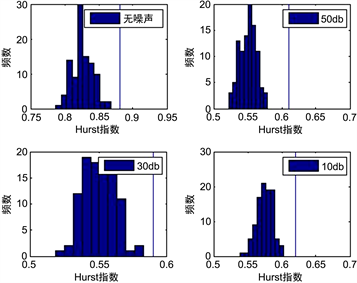(b) Henon映射

Figure 1. Nonlinear testing of chaotic time series with hurst index (The linear representation of the original sequence of the Hurst index)

5. 工程应用实例

5.1. 工程概况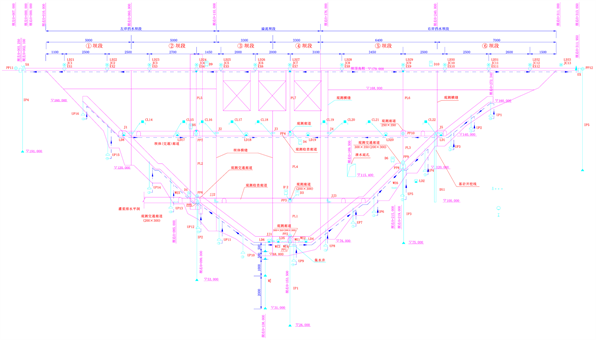Figure 2. Layout of deformation monitoring for a dam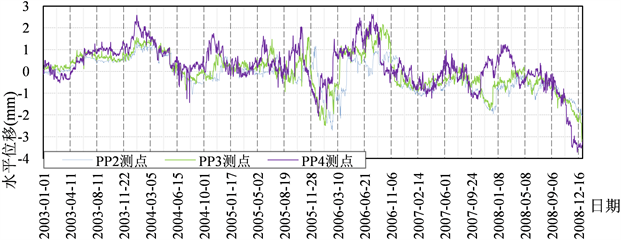Figure 3. Time series of displacement monitoring data of PP2, PP3 and PP4 measuring points

5.2. 计算结果

1) 对三个测点的位移监测数据时间序列在0.05显著性水平下，零假设为原始数据由线性相关随机过程经静态、非线性变换产生，利用IAAFT算法产生100组替代数据，其Sigma检验量计算结果如表2所示。Table 2. The nonlinear test results on time series of displacement monitoring data

2) 采用Hurst指数的替代数据法检验结果如图4所示。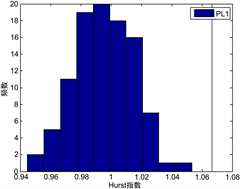(a) PP2测点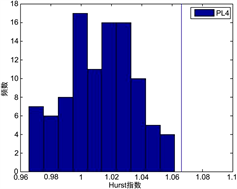(b) PP3测点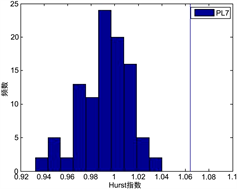(c) PP4测点

Figure 4. Nonlinear testing of chaotic time series with hurst index (The linear representation of the original sequence of the Hurst index)

6. 结论

 江科. 大坝安全监测数据分析方法研究[J]. 科技资讯, 2012(35): 53-54.

 徐洪钟. 大坝动力系统的安全监控非线性分析模型研究[D]: [博士学位论文]. 南京: 河海大学, 2001.

 齐银峰. BP神经网络在大坝变形分析中的应用及改进[D]: [硕士学位论文]. 昆明: 昆明理工大学, 2017.

 卢世坤, 李夕海, 牛超. 基于替代数据法的地磁变化场时间序列非线性检验[J]. 河北师范大学学报: 自然科学版, 2013, 37(6): 32-38.

 李文云. 基于混沌时间序列预测法的岩土施工变形分析[D]: [硕士学位论文]. 昆明: 昆明理工大学, 2016.

 Theiler, J., Eubank, S. Longtin, A., Galdrikian, B. and Farmer, J.D. (1992) Testing for Nonlinearity in Time Series: The Method of Surrogate Data. Physica D: Nonlinear Phenomena, 58, 77-94.
https://doi.org/10.1016/0167-2789(92)90102-S

 Xiao, J., Borgnat, P., Flandrin, P. and Richard, C. (2007) Testing Stationarity with Surrogates—A One Class SVM Approach. 2007 IEEE/SP 14th Workshop on Statistical Signal Processing, Madison, WI, 26-29 August 2007, 720-724.
https://doi.org/10.1109/SSP.2007.4301353

 Xiao, J., Borgnat, P. and Flandrin, P. (2007) Testing Stationarity with Time-Frequency Surrogates. 15th European Signal Processing Conference EUSIPCO-2007, EURASIP, Poznan, Poland, September 2007, 2020-2024.

 谢鲲, 雷敏, 冯正进. 混沌保密通信系统的替代数据检验研究[J]. 信息与控制, 2004, 33(4): 429-433.

 张永东. 上海股票市场非线性与混沌的检验[J]. 管理工程学报, 2003, 17(3): 21-26.

 唐震, 程玉胜. 舰船辐射噪声的非高斯特性和非线性检验[J]. 青岛大学学报: 自然科学版, 2002, 15(3): 63-65.

 徐平. 大坝观测数据的吸引子分析[J]. 大坝观测与土工测试, 1992(5): 44-47.

 刘洪, 李必强. 基于混沌吸引子的时间序列预测[J]. 系统工程与电子技术, 1997(2): 23-28.

 吴浩, 侯威, 王文祥, 颜鹏程. 试用Lyapunov指数探讨气候突变及其前兆信号[J]. 物理学报, 2013, 62(12): 558-565.

 刘德志, 李俊杰. 大坝安全监测资料的非线性检验[J]. 应用基础与工程科学学报, 2006, 14(1): 84-92.

 眭烨. 替代数据及其应用[D]: [硕士学位论文]. 上海: 华东师范大学, 2011.

 卢世坤, 李夕海, 牛超, 曾小牛, 杨晓芸. 一种基于Fisher投影的时间序列非线性检验[J]. 振动与冲击, 2015, 34(5): 179-185.

 朱凯, 张钦杰, 陈晨, 冯庚. 基于小波去噪方法的云模型在大坝裂缝宽度安全监控指标拟定中的应用[J]. 水电能源科学, 2013, 31(4): 62-65+149.

Top